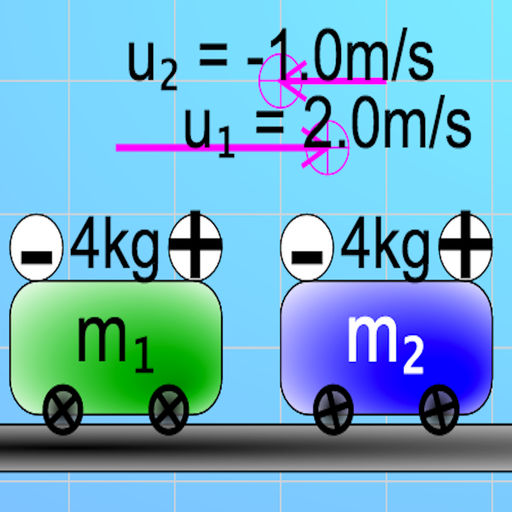Collision Carts Simulator# Collision Carts Simulator

## loo kang wee

Free
0

0 Ratings

Update Date

2019-01-11

Size

25.0 MB

### Screenshots for iPhone

iPhone
Collision Carts Simulator Description
An open source physics at Singapore simulation based on codes written by Loo Kang Wee and Sze Yee Lye.
more resources can be found here
http://iwant2study.org/ospsg/index.php/interactive-resources/physics/02-newtonian-mechanics/02-dynamics

Introduction
Momentum One Dimension Collision Model
The motion of a body of mass m and velocity v is described by a vector quantity known as momentum p where
p = mv
When objects collide, whether trains, cars, billiard balls, shopping carts, or your foot and the sidewalk, the results can be complicated. Yet even in the most chaotic of collisions, as long as there are no net external forces acting on the colliding objects, one principle always holds and provides an excellent tool for understanding the collision. That principle is called the conservation of linear momentum which states that

The total momentum of a system remains constant provided that no external resultant force acts on the system

For two bodies colliding linearly, it is written mathematically as a vector equation

Total initial momentum = total final momentum
m1.u1 + m2.u2 = m1.v1 + m2.v2

If external forces (such as friction) are ignored, the total momentum of two carts prior to a collision (left side of the equation) is the same as the total momentum of the carts after the collision (right side of the equation).
Collisions are classified into elastic (or perfectly elastic), inelastic and completely inelastic.
There is also a concept of the kinetic energy of a moving body is stated mathematically by the following equation:
KE1 = ½ m1.v12
Main Simulation View
The simulation has 2 collision carts on frictionless floor and wheels.
Sliders
Explore the sliders allows varying the variables.
* mass of cart ONE, mass_1, m1 in kg
* initial velocity of cart ONE, u1 in m/s
* mass of cart TWO, mass_2, m2 in kg
* initial velocity of cart TWO, u2 in m/s
Allows for selecting what kind of collision is simulated.
A Perfectly elastic collision is defined as one in which both conservations of momentum and conservation of kinetic energy are observed
A Perfectly Inelastic collision is defined as one in which conservation of momentum is observed but the colliding carts stick together after the collision with kinetic energy loss
show: velocity, for visualizing the velocity vector
plot momentum vs time graph, for different representation of data for the momentum of cart 1, 2 and both.
plot kinetic energy vs time graph, for different representation of data for the kinetic energy of cart 1, 2 and both.
hint: COM, for the equation of conservation of momentum
hint: COKE, or the equation of conservation of kinetic energy
Buttons
Play
Step Forward
Reset
have their usual meaning.

Interesting Fact
This simulation has real and ideal collision simulator targeted for A level Physics education.

Acknowledgment
My sincere gratitude for the tireless contributions of Francisco Esquembre, Fu-Kwun Hwang, Wolfgang Christian, Félix Jesús Garcia Clemente, Anne Cox, Andrew Duffy, Todd Timberlake and many more in the Open Source Physics community.
Collision Carts Simulator 0.0.7 Update
2019-01-11 Version History
optimized for iOS
More
Price:
Free
Version:
0.0.7
Size:
25.0 MB
Update Date:
2019-01-11
Developer:
loo kang wee
Language:
English
More
You May Also Like
Developer Apps